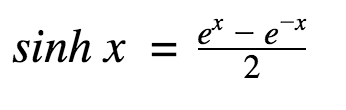# C++ sinh()

#### The sinh() function in C++ returns the hyperbolic sine of an angle given in radians.

The function is defined in <cmath> header file.

`[Mathematics] sinh x = sinh(x) [In C++ Programming]`

## sinh() prototype [As of C++ 11 standard]

```double sinh(double x);
float sinh(float x);
long double sinh(long double x);
double sinh(T x); // For integral type.```

The sinh() function takes a single argument in radians and returns the hyperbolic sine of that angle in `double`, `float` or `long double` type.

The hyperbolic sine of x is given by,## sinh() Parameters

The sinh() function takes a single mandatory argument representing a hyperbolic angle in radian.

## sinh() Return value

The sinh() function returns the hyperbolic sine of the argument.

If the magnitude of the result is too large to be represented by a value of the return type, the function returns HUGE_VAL with the proper sign, and an overflow range error occurs.

## Example 1: How sinh() function works?

``````#include <iostream>
#include <cmath>
using namespace std;
int main()
{
double x = 3.55, result;

result = sinh(x);
cout << "sinh(x) = " << result << endl;

// x in Degrees
double xDegrees = 90;
x = xDegrees * 3.14159/180;

result = sinh(x);
cout << "sinh(x) = " << result << endl;

return 0;
}``````

When you run the program, the output will be:

```sinh(x) = 17.3923
sinh(x) = 2.3013```

## Example 2: sinh() function with integral type

``````#include <iostream>
#include <cmath>
using namespace std;
int main()
{
int x = -3;
double result;

result = sinh(x);
cout << "sinh(x) = " << result << endl;

return 0;
}```
```

When you run the program, the output will be:

```sinh(x) = -10.0179
```